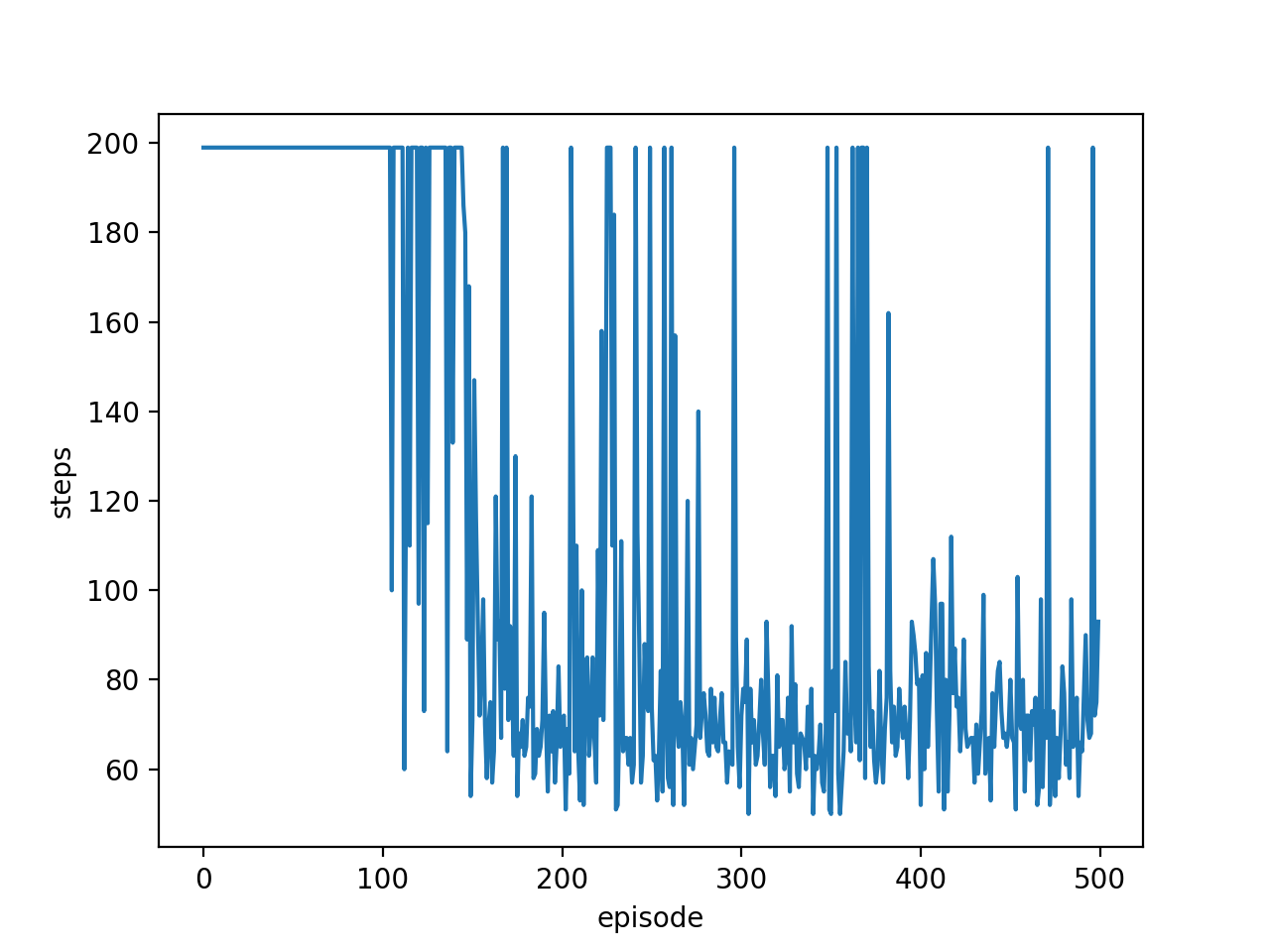# 从头开始做一个机器手臂5 完善测试

• 甩手结束 (需要改环境)
• 不太收敛 (引起这个问题的起因很多)
• 网络性能不好?
• 特征没选好?
• reward 没设好?

## 解决甩手问题 ¶

# env.py

class ArmEnv(object):
def __init__(self):
...
self.on_goal = 0

def step(action):
...
# done and reward
if self.goal['x'] - self.goal['l']/2 < finger < self.goal['x'] + self.goal['l']/2:
if self.goal['y'] - self.goal['l']/2 < finger < self.goal['y'] + self.goal['l']/2:
r += 1.
self.on_goal += 1
if self.on_goal > 50:
done = True
else:
self.on_goal = 0
...


## Reward Engineering ¶

class ArmEnv(object):
def step(self, action):
...
# dist2 是 finger 离 goal 的 x,y方向的距离
dist2 = [(self.goal['x'] - finger) / 400, (self.goal['y'] - finger) / 400]
r = -np.sqrt(dist2**2+dist2**2)
...


## Feature Engineering ¶

# env.py

class ArmEnv(object):
state_dim = 9       # state 的维度变到 9
def step(self, action):
...
a1xy_ = np.array([np.cos(a1r), np.sin(a1r)]) * a1l + a1xy  # a1 end and a2 start (x1, y1)
finger = np.array([np.cos(a1r + a2r), np.sin(a1r + a2r)]) * a2l + a1xy_  # a2 end (x2, y2)
# normalize features
dist1 = [(self.goal['x'] - a1xy_) / 400, (self.goal['y'] - a1xy_) / 400]
dist2 = [(self.goal['x'] - finger) / 400, (self.goal['y'] - finger) / 400]
...
# state
s = np.concatenate((a1xy_/200, finger/200, dist1 + dist2, [1. if self.on_goal else 0.]))
return s, r, done• 增大记忆库 (MEMORY_CAPACITY)
• 减小学习率 (LR_A/LR_C)
• 增大神经网络规模